## Sunday 10 August 2014

### Differential Equations, Summation Series, and Washing Dishes

Suppose you have a glass full of some liquid that you need to get rid of and rinse out so that you can safely drink from the glass again. It doesn't really matter what the stuff is for this analysis, as long as it mixes with water (vinegar, bleach, spoiled milk, soapy water, whatever). We'll call this stuff the “pollutant”. You probably know from experience that it takes a lot of water to get rid of the pollutant if you simply run the tap and let the glass overflow until it's clean (we'll call this the "overflow method"). It's way more efficient to dump the stuff out and rinse the glass, repeating as necessary (we'll call this the "rinse-repeat method"). If you don't believe me, go try it out. Fill a glass with milk or coffee and then run the tap and see how long it takes before the glass is full of clear water.An overflowing glass. Enjoy this picture because there's a lot of math coming up.

### Overflow Method

We know qualitatively that the rinse-repeat method is a much more efficient use of water, but in this post we'll actually quantify the difference. Let's start by analyzing the overflow method. The glass initially has some quantity of pollutant in it, P. If you run the tap and overflow the glass, how much water do you need to reduce the amount of pollutant in the glass to some suitably low value? For instance, if you had a glass full of soapy water, how much water would you need to use to remove 99.9% of the soap from the glass? The problem can be modeled with a first-order differential equation.

Let’s describe the problem in words first. The rate of change in the quantity of pollutant in the glass is equal to the rate of pollutant coming in (in this case zero because we assume pure water is being added) minus the rate of pollutant going out (the concentration of pollutant in the glass multiplied by the flow rate of liquid leaving the glass, q). If we assume perfect mixing, the concentration of pollutant in the glass at any moment in time is equal to the quantity of pollutant in the glass, P, divided by the volume of the glass, VG. Mathematically, the problem can be expressed as:Equation 1

This is a first-order differential equation because the rate of change of P is a linear function of P. The solution to this one is simple as far as differential equations go, and looks something like this: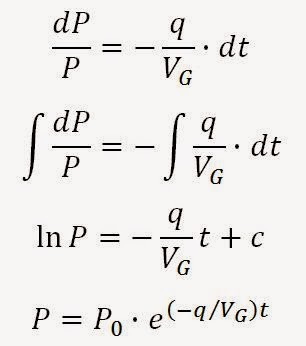Solution to Equation 1

If we divide by P we have an expression for the proportion of pollutant remaining in the glass: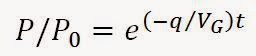Equation 2

We can specify some value of pollutant remaining (say 0.1%) and solve for the amount of time it will take to get there:Rearranging Equation 2 to solve for time t

But we’re looking for the amount of water we’re using, not how long it’ll take. The volume of water that spilled out of the glass is simply equal to tf multiplied by the flow rate q. If the glass wasn't completely full of pollutant to start with, then there is also some amount of water required to reach the onset of overflowing, equal to the difference between VG and P0. Therefore,Equation 3

If we divide by the volume of the glass and replace P0/VG with the initial concentration α0, we get a general expression that will tell us how much water is needed (in multiples of the volume of the glass) to remove the pollutant by the overflow method until you've reached some reduced level Pf/P0.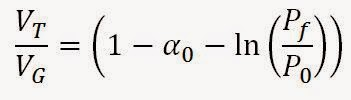Equation 4Dilution water required when using the overflow method to remove a pollutant.

Plotting Equation 4 gives this graph: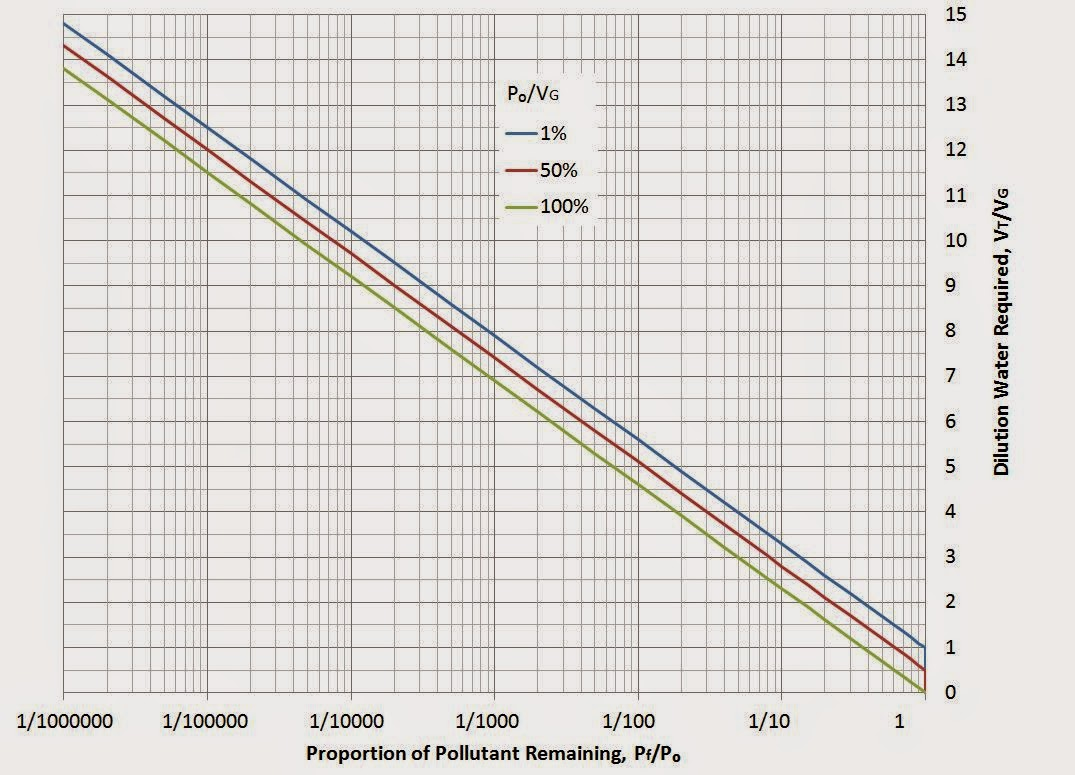Normalized volume of dilution water required to reduce pollutant levels to some threshold Pf/P0

I used a logarithmic scale for the horizontal axis because it makes a nice linear plot. What the plot shows is that to remove 99.9% of the original quantity of pollutant in the glass (Pf/P0 = 1/1000) using the overflow method, you need to run the tap and use an amount of water equal to at least 7 times the volume of the glass.

To give a simple example, suppose your favourite mug (assume 350 mL volume) is half full of old coffee that you want to get rid of. If you just run the tap to make the mug overflow until the mug's clean, how much water would you be using? To remove 99.9% of the 175 mL of old coffee (i.e. 0.175 mL left in the mug), you would have to use almost 2,600 mL of water.

### Rinse-Repeat Method

Now for the rinse-repeat method. Let’s assume that after dumping out the contents of the glass there is some residual volume left Vresid, which can also be expressed as some proportion of VG, i.e.: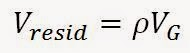Residual volume in the glass after dumping the contents

Let’s also assume that after dumping the contents, you add some pure water to rinse out the glass, again some proportion of VG:Volume of rinse water per rinse cycle

If we start with some volume of pollutant P0, upon dumping the contents the first time, before any rinsing is done, there is ρVG pollutant remaining in the glass. Then ηVG is added, diluting the residual pollutant to a concentration of:Concentration of pollutant in glass after first rinse cycle

After dumping that diluted liquid out from the first rinse, you’re left with some residual pollutant in the glass, equal to:Residual pollutant after one rinse cycle

Repeating the cycle a 2nd and 3rd time would leave residual amounts of pollutants equal to: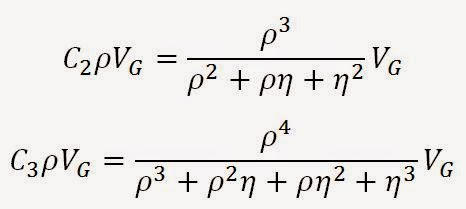Residual pollutant after two and three rinse cycles

In general, the residual pollutant after emptying the glass after rinsing for the Nth time is:Equation 5Residual pollutant after N rinse cycles

The summation term isn't convenient to work with, but luckily there is a closed form solution to this sum and we can express Equation 5 as:Equation 6Alternate expression for the residual pollutant after N rinse cycles

Now we’re interested in reducing the amount of pollutant to some threshold level of Pf/P0. Equating Pf  to Equation 6 and then rearranging:Equation 7Proportion of pollutant remaining after N rinse cycles

Solving Equation 7 for N is a little tricky, but it can be done. We get:Equation 8Number of rinse cycles N necessary to reduce pollutant levels to some threshold Pf/P0

We also could have made a useful approximation to make it easier to solve for N. Since ρ is probably much smaller than η, we can approximate Equation 7 with the following:Useful approximation of Equation 7 when ρ << η

Which is accurate to within 10% for ρ/η < 1/10. Solving for N from the approximate expression:Approximation of the number of rinse cycles N necessary to reduce pollutant levels to some threshold Pf/P0

Based on how we've defined the problem, N technically has to be an integer, but we can ignore that detail for the purpose of estimating the amount of dilution water required. If we rinse N times, the total amount of water used to remove the pollutant is simply:Dilution water required to remove the pollutant using the rinse-repeat method

Normalizing as before, we get Equation 9:Equation 9Dilution water required to reduce pollutant levels to some threshold Pf/P0 using the rinse-repeat method

After all that math, what do we have (besides a headache)? Well we can make a graph like we did for the overflow method, shown below:

It's clear from these plots that the rinse-repeat method is far more efficient at removing the pollutant. To illustrate, let's go back to the example of the 350 mL mug initially containing 175 mL of old coffee. Assuming ρ = 0.01 and η = 0.5, after just one cycle we've removed almost 99.99% of the pollutant. That's only 175 mL of dilution water and we already have an order of magnitude improvement in pollutant removal compared to the overflow method using almost 15 times as much water. This chart below shows just how much of a difference there is between the two methods: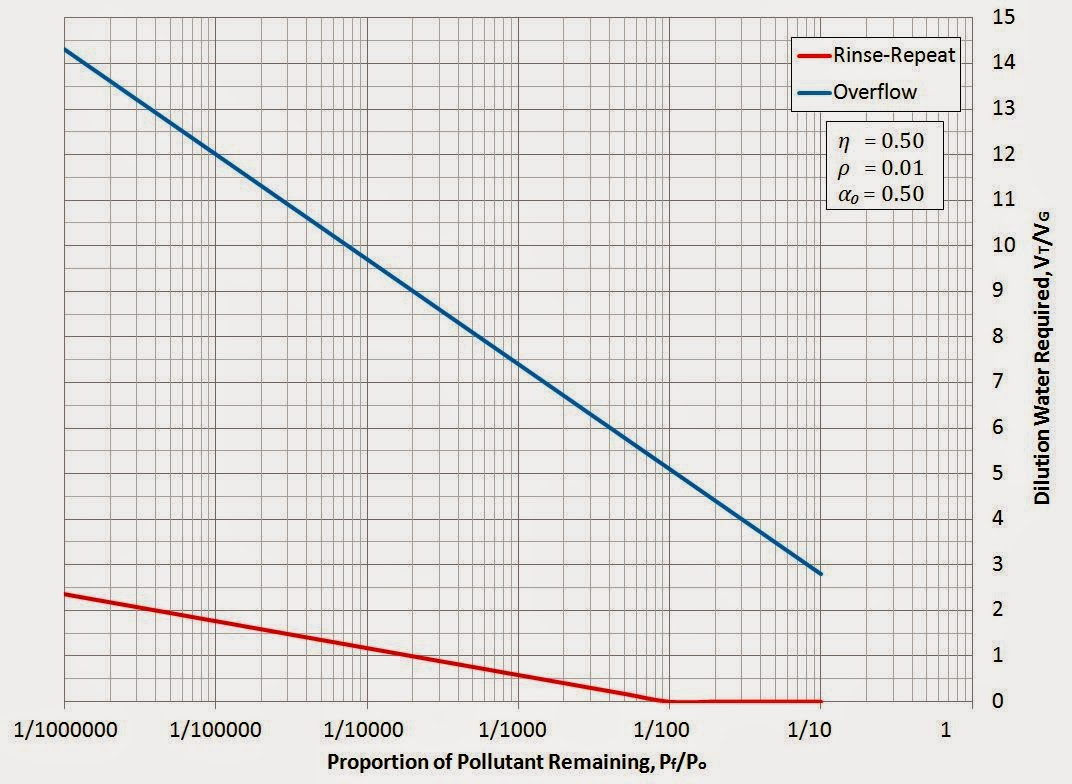Comparison of the two pollutant removal methods.

The overflow method requires about 12 times more water to remove 99.9999% of the stale coffee in our example. If we go to a completely unreasonable level of pollutant removal, beyond even the dilution scale of homeopathic "medicine" preparations, the overflow method still wastes way more water. You can see for yourself in this table below:Comparison of the overflow and rinse-repeat methods for the coffee mug example.

Of course, at extreme dilution levels, we eventually get down to the situation where it becomes statistically unlikely that a single molecule of the original substance is left, so at these levels my equations don't actually make meaningful predictions. But it still effectively illustrates that there is no situation where the overflow method is a more efficient use of water. It doesn't even come close.

In conclusion, overflowing a cup or bowl to clean it is absurdly wasteful of water. You already knew that, but now you've seen the math to back it up.